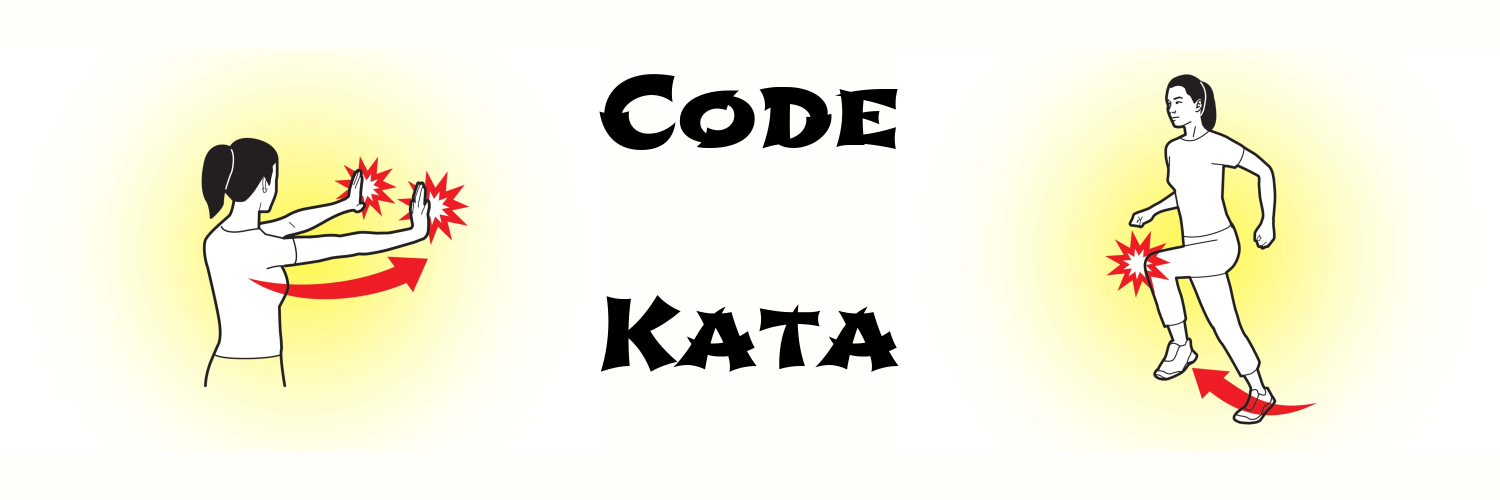# Programming Kata Day 8# Problem

Write a function steps, that takes a sequence and makes a deeply nested structure from it:

(steps [1 2 3 4])
;=> [1 [2 [3 [4 []]]]]


# Solution 1

(defn steps [s]
(if (seq s)
[(first s) (steps (rest s))]
[]))


# Solution 2

Lazy version:

(defn lz-steps [s]
(lazy-seq
(if (seq s)
[(first s) (lz-steps (rest s))]
[])))


# Note

To see the difference, call the function like this:

(take 1 (steps (range 10000000)))

(take 1 (lz-steps (range 10000000)))